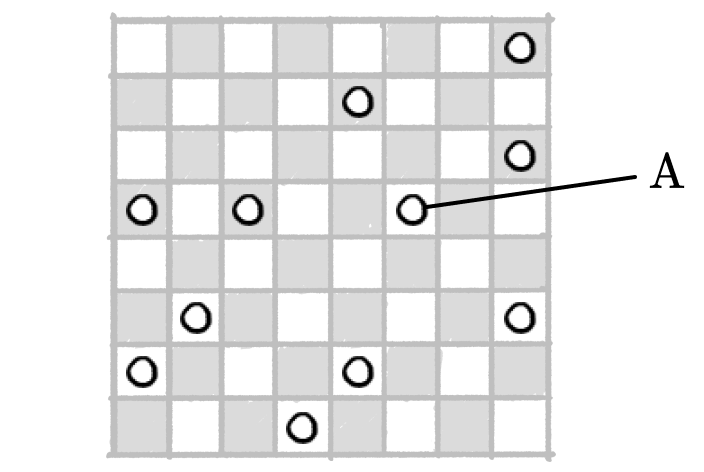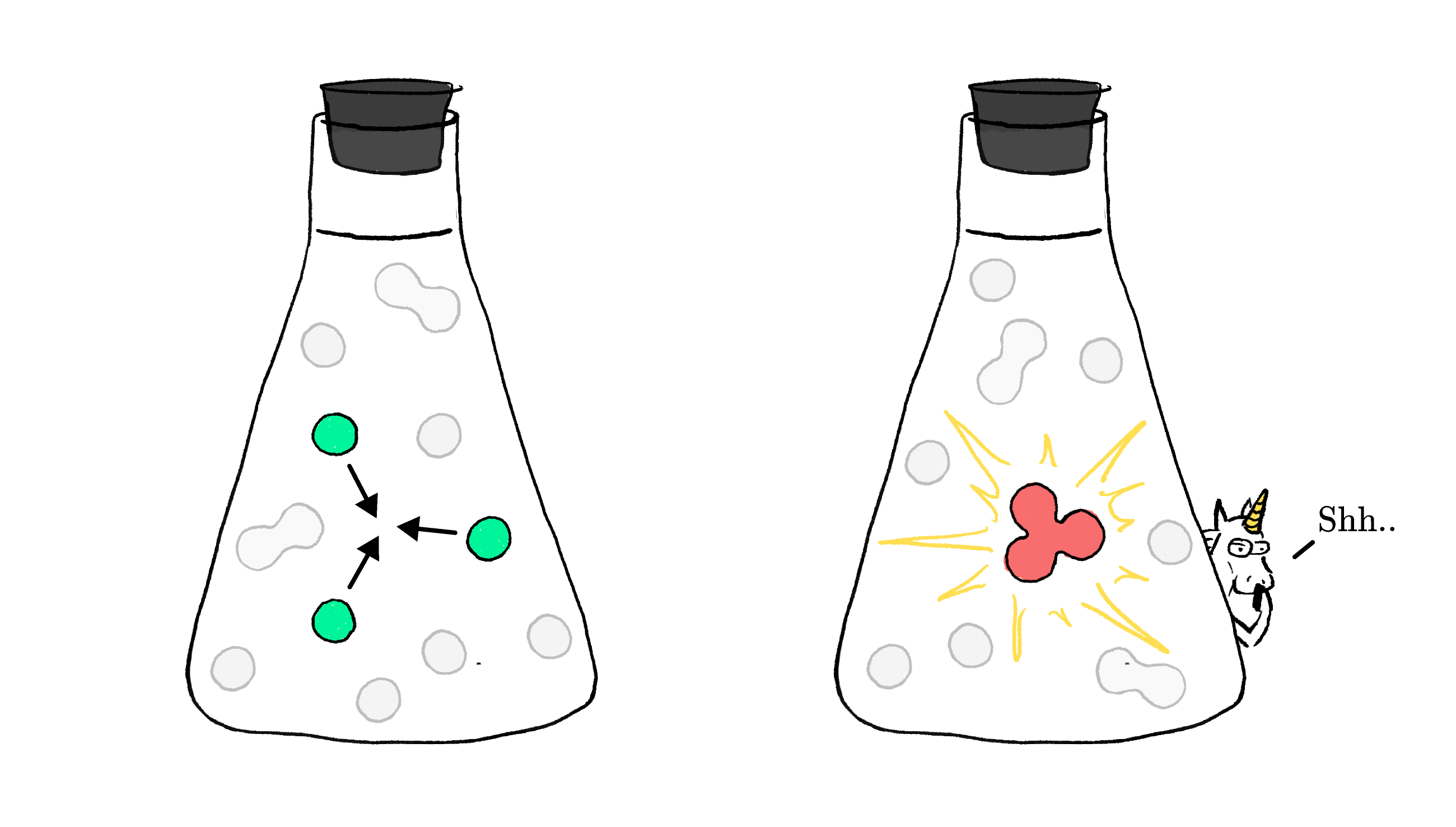### The Chemical Reaction

Chemistry is all about the exciting ways that molecules can change and transform.

Sometimes a molecule can "react" on its own and break into pieces — but more often than not, chemical reactions occur between two or more molecules. In order for them to react, they first need to find one another.

The time it takes for a reaction to occur depends on the details. If molecules are few and far between, or if the reaction needs many molecules to collide at once, the reaction will take longer to happen.

In this exploration, we're going to uncover the link between chemical reactions and probability to benchmark the kinetics of fundamental reactions.

# Molecular Choreography

A few things need to happen for a chemical reaction to take place between molecules.

If two or more molecules are going to react, then, first of all, they need to collide with sufficient energy and make contact in just the right orientation.

But for a reaction to occur at all, the molecules have to be in the same location.

# Molecular Choreography

Most reactions take place in a liquid or gas confined to a flask of fixed volume, and a molecule can be at any position within it.

We're going to make things as simple as possible and assume the flask is like a chessboard, with a fixed number of positions $V$ that the $N$ molecules can occupy.To get started, let's monitor the action at a position we'll call Site A. If we assume that the $N$ sugar molecules are randomly distributed throughout the flask, what is the probability that one sugar molecule is at Site A?

# Molecular Choreography

The probability that a molecule is at Site A is just the number of molecules $N$ divided by the number of positions it can be in, $V:$

$p = \dfrac{N}{V}.$

This probability also goes by a more common name: concentration. The higher the concentration of a molecule is in a flask, the more molecules there are per unit volume. If we want a more concentrated sugar solution, we just add more sugar molecules to the solution without changing the amount of water.

Usually, both the number of molecules and the number of locations in a flask are such enormous numbers that they’re not useful to think about directly; the ratio is much more practical. The concentration of a molecule $\ce{B}$ is represented by

$\ce{[B]} = \frac{N_B}{V}.$

# Molecular Choreography

In order for two molecules to react with each other, they have to be at the same position at the same time.

Concentration tells us the probability that one molecule of a particular type is at a particular location — it doesn't tell us anything about where any given molecule is, and it doesn't change unless we change the number of molecules in the flask, or change the volume.

What is the probability that a molecule of $\ce{B}$ and a molecule of $\ce{C}$ are both at Site A?

# Molecular Choreography

Let's be clear: there’s nothing special about Site A! If the molecules are randomly distributed, then every location in the flask is equivalent to any other.

But we said that molecules have to collide in order to react. To find the total number of collisions that happen each moment, we need to know the probability for a collision at any given position as well as the total number of positions.

Suppose there are $500$ molecules of $\ce{B}$ and $500$ molecules of $\ce{C}$ randomly distributed among $1000$ locations in the flask. How many collisions do you expect to occur in the first moment if the molecules are truly randomly distributed?

# Molecular Choreography

Let's take this logic one step further! In that first moment, how many reactions between molecules of $\ce{B}$ and $\ce{C}$ do you expect to occur?

# Molecular Choreography

Not all reactions are created equal! If we set up two different reactions and put their reactant molecules at the same concentrations, it's possible for one to proceed very quickly while the other proceeds very slowly.

This is because a collision is only one part of a successful reaction! The other piece of it is ensuring that the molecules collide with enough speed and in the right orientation to cause the transformation.

While we can think about the number of collisions in a purely probabilistic way, the details of successful reactions depend on the speed of the collision and quantum mechanics.

# Molecular Choreography

Nevertheless, we can capture this information in a single number called the rate constant $k,$ a gauge of how likely it is that a collision between two molecules will result in a reaction. The number of reactions that happen between molecules $\ce{B}$ and $\ce{C}$ per second is

$(\text{Reactions per second}) = k \times\ce{[B]}\times \ce{[C]}.$

Some reactions, like the combination of two reactive molecules to form the explosive TNT, will happen with the slightest collision, while the reaction between styrene and propylene to form a plastic polymer requires many collisions before one succeeds.

# Molecular Choreography

Suppose we use the same conditions to run a two-molecule reaction between $\text{B}$ and $\text{C},$ and the reaction proceeds at a rate $r = k\times\ce{[B]\times[C]}.$ We then run the same reaction (with the same number of molecules), but in a flask with $10$ times as much water.

What do you expect to happen to the reaction rate in this new setup?

# Molecular Choreography

As one more example before we go, suppose a reaction requires three molecules of $\ce{A}$ to come together to produce a trimer.How would the rate of the reaction, $r,$ depend on $k$ and $\left[\ce{A}\right]?$

# Molecular Choreography

In this exploration, we used the simple model of a chessboard to better understand how the likelihood of a chemical reaction between two molecules can be described in a quantitative way.

There are other kinds of reactions, though. A single molecule can break apart into many, molecules can be in such large excess that the concentration effectively doesn't matter, and (much more rarely) three or more molecules may come together to react. In all these cases, the same rules apply, but how we envision the rate expression will be slightly different.

There are also many reactions that happen in multiple steps. In those cases, the rate expressions can be even more complex and interesting!

# Molecular Choreography

×